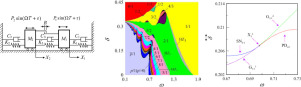International Journal of Mechanical Sciences ( IF 4.134 ) Pub Date : 2020-03-12 , DOI: 10.1016/j.ijmecsci.2020.105605
Xiaohong Lyu; Quanfu Gao; Guanwei LuoA two-degree-of-freedom mechanical impact oscillator with a clearance is considered. Pattern types, occurrence and stability domains and bifurcation characteristics of periodic motions are investigated through two-parameter co-simulation analysis. With varying of the bifurcation parameters in two directions, forward and backward, two-parameter grazing, period-doubling and saddle-node bifurcations of impactless, fundamental and subharmonic motions are calculated and analyzed. There exist two types of transition zones, hysteresis and liguliform zones, in mutual transition between neighboring motions p/1 and (p + 1)/1 (p ≥ 0). Dynamics in the hysteresis and liguliform zones is introduced in detail. Attracting domains and Poincaré mapping diagrams of coexisting motions in the neighborhood of grazing bifurcations are discussed. Pattern types and bifurcations of subharmonic motions in liguliform zones show regularity. In the two-parameter plane, two grazing bifurcation curves of p/1 (p ≥ 0) motion and period-doubling and saddle-node bifurcation curves of (np + 1)/n (n ≥ 1) motion intersect at a singular point along the upper boundary of liguliform zones LZp/1∩(p + 1)/1. Consequently, the singular point is a point of double-grazing bifurcation of p/1 motion and codimension-2 flip-fold bifurcation of (np + 1)/n motion. The grazing bifurcation of neighboring motions is continuous and reversible only at the singular points, and displacement amplitudes of the impact oscillator vary continuously when the varying parameter passes through the grazing bifurcation boundary.

down
wechat
bug